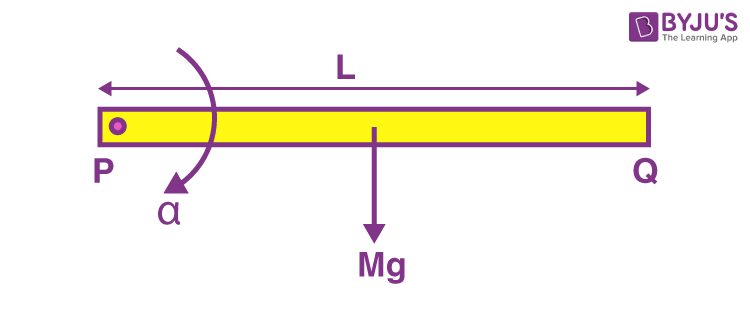# A rod PQ of mass M and length L is hinged at end P. The rod is kept horizontal by a massless string tied to point Q as shown in figure. When string is cut, the initial angular acceleration of the rod is.(a) 2g/L (b) 2g/2L (c) 3g/2L (d) g/LIf is moment of inertia of the rod about the end P and α is initial angular acceleration of the rod, then;

τ=Iα=Mg x L/2

Since,

I = ML2/3

Hence,

ML2/3 α = Mg x L/2

α = 3g/2L